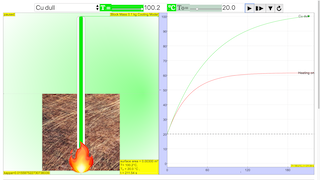Intro Page

image of

copper shiny https://c1.staticflickr.com/1/164/362133253_77585f5429_z.jpg?zz=1

copper dull https://www.colourbox.com/preview/10760507-196481-golden-copper-shiny-abstract-vertical-background.jpg

al shiny http://preview.cutcaster.com/cutcaster-photo-100709683-metal-texture.jpg

al dull http://pixabay.com/p-432524/?no_redirect

fe shiny http://www.burningwell.org/gallery2/d/11247-6/img_0571.jpg

### Translations

Code Language Translator Run### Software Requirements

SoftwareRequirements

 Android iOS Windows MacOS with best with Chrome Chrome Chrome Chrome support full-screen? Yes. Chrome/Opera No. Firefox/ Samsung Internet Not yet Yes Yes cannot work on some mobile browser that don't understand JavaScript such as..... cannot work on Internet Explorer 9 and below

### CreditsThis email address is being protected from spambots. You need JavaScript enabled to view it.; christian wolfgang

### end faq

http://iwant2study.org/lookangejss/03thermalphysics_11thermalpropertiesofmatter/ejss_model_cooling/cooling_Simulation.xhtml

[text]

## Newton's Law of Cooling

The Newton's Law of Cooling model computes the temperature of an object of mass M as it is heated or cooled by the surrounding medium.

### Assumption:

The model assumes that the temperature T within the object is uniform.

### Validity:

This lumped system approximation is valid if the rate of thermal energy transfer within the object is faster than the rate of thermal energy transfer at the surface.

### Convection-cooling "Newton's law of cooling" Model:

Newton assumed that the rate of thermal energy transfer at the object's surface is proportional to the surface area and to the temperature difference between the object and the surrounding medium.

$$\frac{\delta Q}{\delta t} = h A( T(t) - T_{background} )$$

$$Q$$ is the thermal energy in joules
$$h$$ is the heat transfer coefficient (assumed independent of T here) ($$\frac{W}{m^{2} K}$$)
$$A$$ is the heat transfer surface area ($$m^{2}$$)
$$T$$ is the temperature of the object's surface and interior (since these are the same in this approximation)
$$T_{background}$$ is the temperature of the surrounding background environment; i.e. the temperature suitably far from the surface is the time-dependent thermal gradient between environment and object.

### Definition Specific Heat Capacity:

The specific heat capacity of a material on a per mass basis is

$$Q = mc ( T_{final} - T_{initial} )$$
$$Q$$ is heat energy
$$m$$  is the mass of the body
$$c$$ specific heat capacity of a material
$$T_{final}$$ is the $$T_{background}$$
$$T_{initial}$$ is the $$T(t)$$

combing the 2 equations

$$\frac{mc ( T_{background}- T(t) ) }{\delta t} = h A( T(t) - T_{background} )$$

assuming mc is constant'

$$mc \frac{ \delta ( T_{background}- T(t) ) }{\delta t} = h A( T(t) - T_{background} )$$

assuming $$T_{background}$$ is a infinite reservoir

$$\frac{ ( T_{background}) }{\delta t} = 0$$
therefore
$$mc \frac{ ( \delta (- T(t)) ) }{\delta t} = h A( T(t) - T_{background} )$$

negative sign can be taken out of the differential equation.

$$mc \frac{ (\delta T(t) ) }{\delta t} = -h A( T(t) - T_{background} )$$

$$\frac{ ( T(t) ) }{\delta t} = -\frac{h A}{mc }( T(t) - T_{background} )$$

let $$\kappa = \frac{h A}{mc }$$

$$\frac{ ( T(t) ) }{\delta t} = -\kappa ( T(t) - T_{background} )$$

$$heating = \frac{\delta Q}{\delta t} = mc ( \frac{\delta T}{\delta t})$$

the final ODE equation looks like

$$\frac{ ( T(t) ) }{\delta t} = -\kappa ( T(t) - T_{background} ) + \frac{heating}{mc}$$

### Definition Equation Used:

$$V = \frac{m}{\rho}$$

$$V$$ is volume of object
$$\rho$$ is density of object

$$A = 6 (\frac{m}{\rho})^{\frac{2}{3}}$$

$$A$$ surface area of object

assumption of increased surface are

$$A_{increased surface area due to fins} = (2)(6) (\frac{m}{\rho})^{\frac{2}{3}}$$

copper shiny $$c_{Cu}$$ = 385  $$\frac{J}{kg K}$$
$$\rho_{Cu}$$ = 8933  $$\frac{kg}{m^{3}}$$
heat transfer coefficient  $$h_{Cu}$$ = 400 $$\frac{W}{(K m^{2})}$$

copper dull $$c_{Cu}$$ = 385  $$\frac{J}{kg K}$$
$$\rho_{Cu}$$ = 8933  $$\frac{kg}{m^{3}}$$
heat transfer coefficient  $$h_{Cu}$$ = 200 $$\frac{W}{(K m^{2})}$$

aluminium shiny $$c_{Al}$$ = 903  $$\frac{J}{kg K}$$
$$\rho_{Al}$$ = 2702  $$\frac{kg}{m^{3}}$$
heat transfer coefficient  $$h_{Al}$$ = 400 $$\frac{W}{(K m^{2})}$$

aluminium dull $$c_{Al}$$ =  903  $$\frac{J}{kg K}$$
$$\rho_{Al}$$ = 2702  $$\frac{kg}{m^{3}}$$
heat transfer coefficient  $$h_{Al}$$ = 200 $$\frac{W}{(K m^{2})}$$

iron shiny $$c_{Al}$$ = 447  $$\frac{J}{kg K}$$
$$\rho_{Al}$$ = 7870  $$\frac{kg}{m^{3}}$$
heat transfer coefficient  $$h_{Al}$$ = 400 $$\frac{W}{(K m^{2})}$$

iron dull $$c_{Al}$$ =  447  $$\frac{J}{kg K}$$
$$\rho_{Al}$$ = 7870  $$\frac{kg}{m^{3}}$$
heat transfer coefficient  $$h_{Al}$$ = 200 $$\frac{W}{(K m^{2})}$$

Users can select the mass of the object and the material and the model computes the surface area assuming a cubic shape. The model plots the object's temperature as a function of time as the user heats and cools the object. A data-tool button on the temperature graph allows users fit the data to analytic functions.

Note: A typical (rough) heat transfer coefficient h for still air and iron is 6 W/(K m^2) and 400 W/(K m^2) . The Newton's Law of Cooling model assumes h=400 for all shiny and h=200 for dull materials. The actual value of h depends on many parameters including the material, the fluid velocity, the fluid viscosity and the condition of the object's surface.

## References:

1. "Measuring the Specific Heat of Metals by Cooling," William Dittrich, The Physics Teacher, (in press).

## Credits:

1. The Newton's Law of Cooling model was created by Wolfgang Christian using the Easy Java Simulations (EJS) version 4.2 authoring and modeling tool.
2. EJSS Cube Block Cooling Model was created by Wolfgang Christian and recreated by lookang using the Easy Java Simulations (EJS) version 5.1 authoring and modeling tool

Research

[text]

[text]

### Hands-On Kits

1. http://www.addest.com/products/category/Science_Kits by Addest StationCooling Curve Kit

[text]

### end faqRating 5.00 (1 Vote)